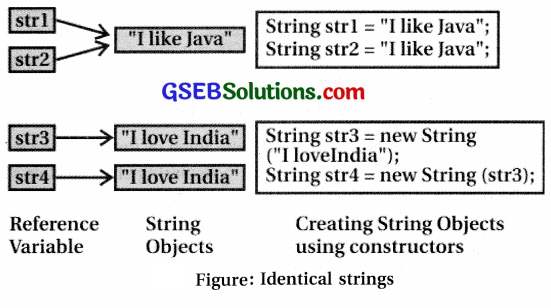# Computer Class 12 GSEB Solutions Chapter 9 Working with Array and String

Students frequently turn to Computer Class 12 GSEB Solutions and GSEB Computer Textbook Solutions Class 12 Chapter 9 Working with Array and String for practice and self-assessment.

## GSEB Computer Textbook Solutions Class 12 Chapter 9 Working with Array and String

Question 1.
Explain array giving example.

• An array is a variable representing a collection of homogeneous type of elements.
• Arrays are useful to represent vector, matrix and other multi-dimensional data.
• Vector is one dimensional (1-D) data structure that can be used to store list of items like characters, numbers.
• Matrix is used to represent two dimensional (2-D) data structures like table or rows and columns.
• Arrays are useful when same operations are to be performed on various elements of the similar type.
• All the elements of an array are stored in memory using contiguous storage space.
• Each element is identified by an index position associated with array variable. In Java, array is an object used to manage list of items.
• Creating an array is a two step process :
1. Declare an array object
2. Create an array object
• An array object can be created in two ways :
1. Using new operator and specifying the size.
2. Directly initializing the content of arrayQuestion 2.
Differentiate between 1-D and 2-D arrays.
1-D Array

• Array with single dimension is known as 1-D array. For example, vector can be seen as a collection of one or more scalar variables.
• Instead of declaring individual variables like marksl, marks2, marks3, marks4, marks5; one can declare array marks  and accesses individual variable elements as marks, marks, marks, marks, marks,
• To declare a 1-D array a pair of square brackets [ ] is used after array name or after data type.
• The syntax to declare array is as follows :
<data typexarray name> [ ]; or
<data typo [ ] <array names ;
• For example, to store the marks obtained by a student in five tests in Mathematics subject, an array of five integer elements can be used.
• Array object named ‘marks’ with size for 5 elements can be created as follows :
int marks[ ] // declare array object
marks = new int; // create array object
• The above two steps can be combined to create a single statement as follows :
int marks[ ] = new int ; or
int[ ] marks = new int;
• Here the name of the array is ‘marks’. It refers to memory location where five integer values are stored.
• Integer of int data type uses 4 bytes storage space. Thus array ‘marks’ requires 5 × 4 = 20 bytes in contiguous locations in memory.
• To refer an element of an array, an index or subscript is used in square bracket [ ] after array variable name as shown in figure.
• Index specifies the position of an element in an array; for example, marks.
• Index value starts from 0.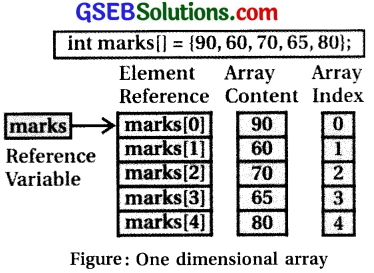• In figure the array variable marks has an index value from 0 to 4.
• Here, marks  refers to the first element and marks refers to the last element.
• As explained in the previous chapter, an object is a reference to variable.
• Array being an object in java, array name is also a reference variable.
• It contains reference to memory location where the array elements are stored, (can be seen in figure).
• As array is an object, so its elements are initialized with default values.
• Figure shows an example of how to use an array in java programs.• One can also specify the initial values of data elements while declaring an array.
• 1-D array is initialized using comma separated values of data elements in braces { }.
• For example :
int marks[ ] = {90, 70, 77}; or
int [ ] marks = {90, 70, 77};
• Note that when an array is initialized while creating, it does not need the use of new operator.
• Its size is same as the number of values specified in braces.
• The code shown in figure uses different ways to create and initialize array.
• Note the use of class method display}) used to display contents of an array.
• Class methods are declared as static and can be referred without any object in the class.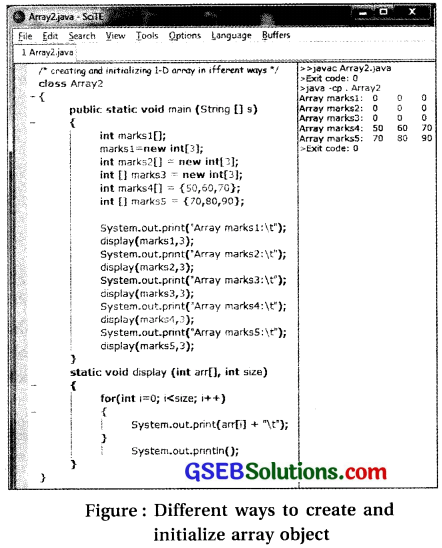• In Java, we cannot specify both the size of dimensions and initial values of array elements simultaneously, while an array declaration.
• Figure shows the code to illustrate the statement.
•  If we declare an array variable without initialization, only a variable is created to hold the reference as shown in figure.
• It does not create an array object, i.e. no memory is allocated for array elements.
• If the elements of an array are accessed, it will result in an error.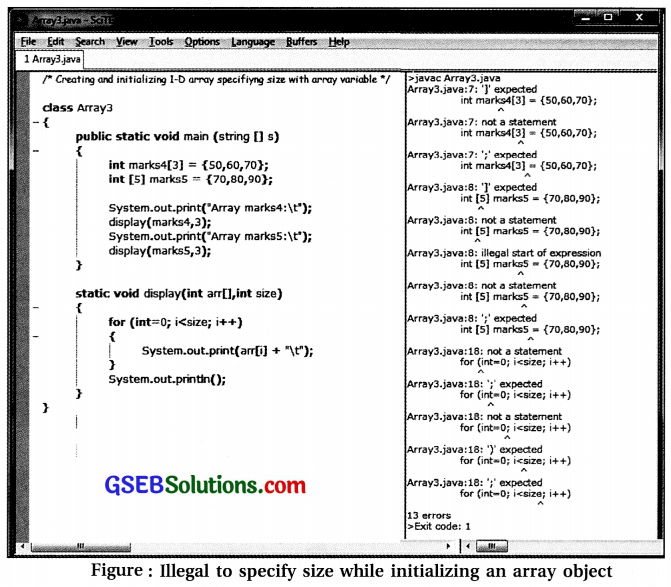• Every element of an array is an individual variable that can be referred by using index.
• Like variables, to change the value of an element, an assignment statement can be used which refers a variable element on left side. For example, marks  = 56; Program in figure computes an average of 10 floating point numbers and executes it.• Observe the code listing in figure and its output. Here there is a need to use the same operation ‘add number to sum’ repeatedly for different elements of same type.
• Use of an array helps in declaring 10 variables at a time and using a loop to perform same operation on different variable elements of an array. Many other types of operations can be performed on array.
• For example, compare two arrays, copy all the elements of one array to another, and search for a specified element in array, sort elements of array and so on. For all such operations, procedures have to be written in the similar way as for finding average of elements.
• Instead of writing the code, various static methods provided by Java using java.util.Arraysclass can be used. In Java, array is treated as an object of this Arrays class.
• This enables us to make use of methods of Arrays class with array type of data. The code listing and its output in figure shows how to use methods sort() and fill0 of java.util.Arrays class. To sort an entire or part of array, sort() method can be used.
• When an array is used as an argument, it sorts an entire array. When this method is invoked with 3 arguments: array, start, last; it sorts partial array from element at index start to element at index (last-1).
• For example, the method call java.util.Arrays.sort(list, 1, 5) sorts elements list from listjl] to list[5-l]; Observe figure.
• Method ‘fill’ is used to fill or partial array with specified value. When the method is invoked with two arguments, array and value; it assigns the specified value to all array elements. When it is invoked with four arguments, array, start, last, value; it fills partial array from element at start to (last-1) with specified value.
• For example, fill (list, 7) assigns value 7 to all elements of list array; Whereas fill (list, 2, 6, 5) assigns value 5 to elements list  to list [6-1]. Observe figure.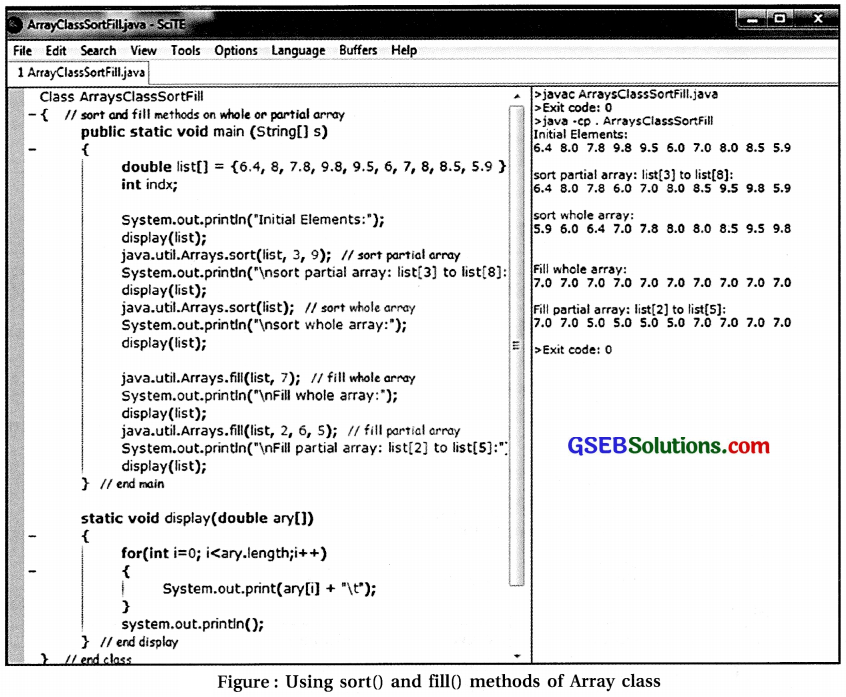• To search an element in an array, Arrays class provides binarySearchO method. Linear search method does element by element comparison in a serial manner. Refer code listing and its output given in figure. It returns index position when an element is found; otherwise it returns -1.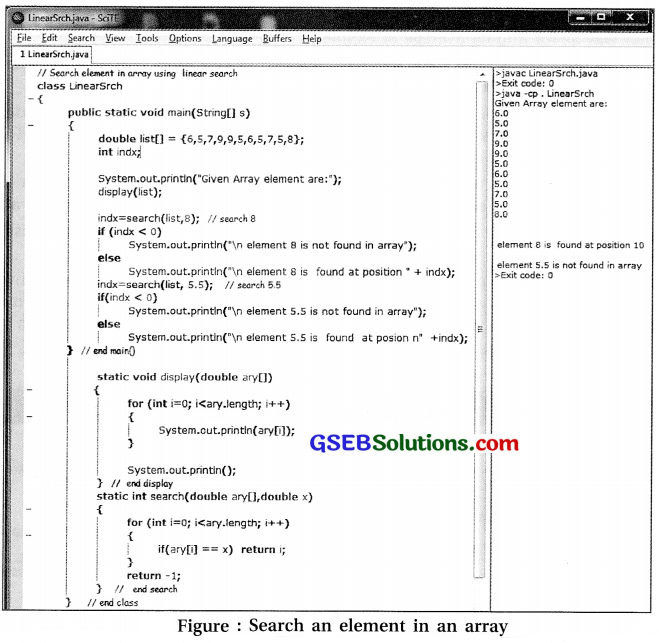2-D Array

• Two dimensional (2-D) arrays are used to store tabular data in the form of rows and columns.
• For example, to store 5 student’s marks in 3 tests, one can use a tabular arrangement of marks in 5 rows and 3 columns as given in figure.
• In mathematics, it is called as a matrix of 5 rows and 3 columns where each element contains marks.
 Students Test1 Test2 Test3 1 50 60 70 2 35 30 50 3 70 75 80 4 80 85 90 5 40 50 55

Figure : Tabular representation of 2-D array

• In Java, 2-D array can be declared using array name and 2 pairs of square brackets [ ] [ ], to specify the size of two dimensions row and column respectively.
• For example, following statement declares and creates an array named marks to store 5 x 3 – 15 integer values in contiguous memory locations.
int marks [ ] [ ] = new int   ;
• Here, the logical Hew of array elements is a table of 5 rows and 3 columns.
• Physically, they are stored in memory using contiguous memory locations for 15 integers that is 60 bytes.
• Java does not support multi-dimensional arrays directly.
• To create 2-D array, an array of an array has to be created. There is no limit on number of dimensions.
In marks  , it creates 1-D array of 5 elements -and each element is 1-D array object of 3 integers as shown in figure.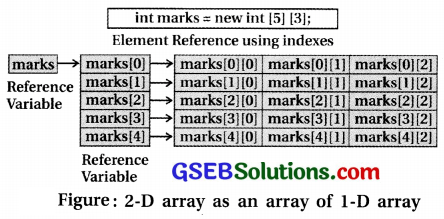• Declaration and initialization of 2-D array is very similar to 1-D array except the number of dimensions. In 2-D array, each row is considered as 1-D array element.
• Thus, like 1-D array initialization, each row is initialized by enclosing its elements in a pair of braces { } separated by comma.
• To initialize 2-D array, all these initialized rows are to be enclosed in curly braces { } separating them by comma (, ). Like 1-D array, 2-D arrays can be declared in various ways as seen in code listing given in figure .
• Figure shows output of the code.• In Java, 2-D array is considered as an array of 1-D array. So, rows of 2-D array can have variable
number of columns.
• Figure shows how to use 2-D array of characters to store five names of computer programming languages.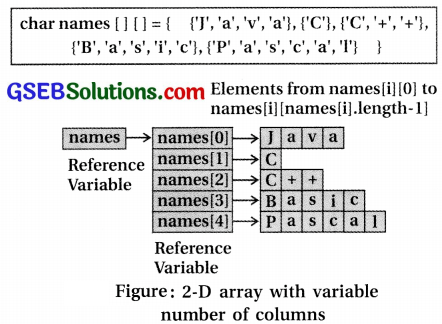• Each name is stored in different row and all names have different number of characters.
• Thus each row is of different size.
• Size of each row can be known using ‘length’ property of 1-D array
• Figure shows how to use 2-D array with varying column size.
• Here length’ property has been used 10 get the number of elements in 1-D array.
• For 2-D array, it returns number of rows.
• For 1-D arraY it returns number of columns in specified row element.
• For 2-D array. number of elements is considered
as number of rows and each row is 1-D array.
• In short, length property used with only array name returns size of its first dimension.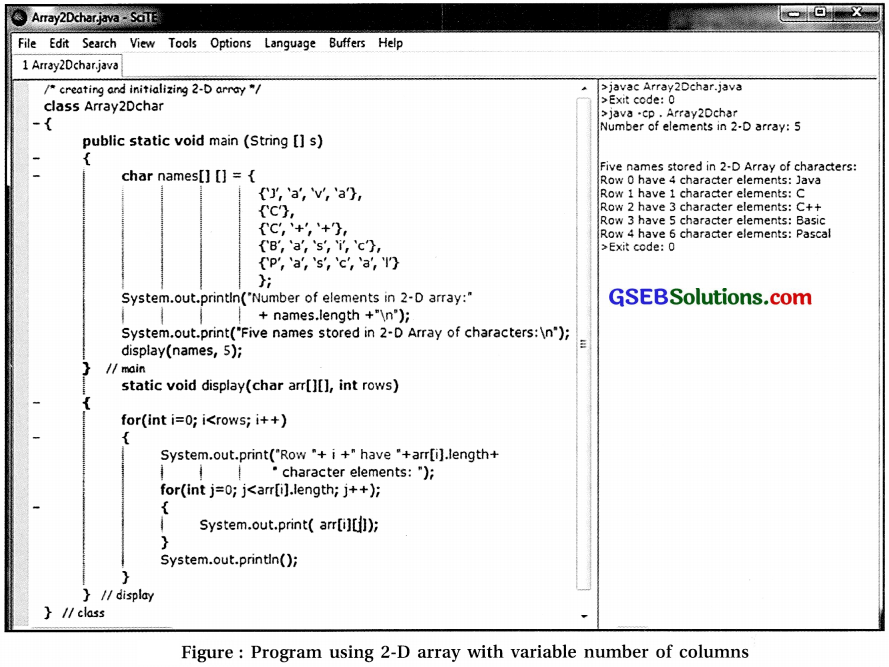• In figure, 2-D array of bytes has been used to store names.
• It shows that initial char type data values are converted to byte data type and the characters of names are assigned their corresponding ASCII values.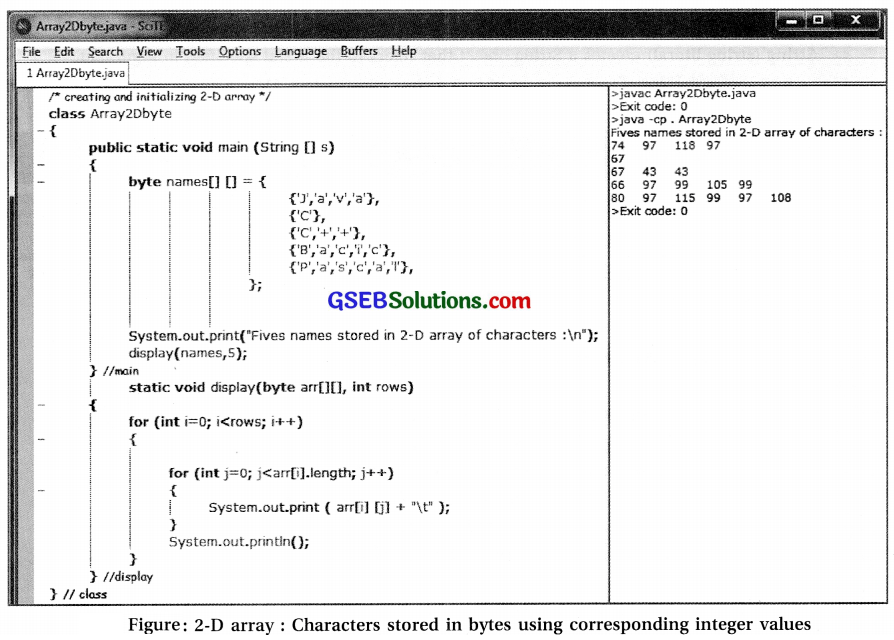Points to be remembered :

1. Array is a collection of homogeneous type of data.
2. Array elements can be accessed using index for each dimension in [ ].
3. Index value starts with zero.
4. Multi-dimensional arrays can have variable size of 2nd, 3rd … dimensions.
5. Attribute ‘length’ is used to determine the size of the dimension.
6. Array is treated as an object.
7. Declaring an array without initialization does not create an array object.

Question 3.
Explain the use of the following classes :
(a) String
(b) Date
(c) Calendar
(a) String

• The String class provides methods to compare strings, find length of string, combining strings, obtaining substrings, converting strings, splitting strings, searching for character or patterns in string etc.
• The program in figure shows different ways to compare strings or part of strings.• The syntax and description of various comparison methods is shown in table 9.1
 Method Description boolean equals (String str) Returns true if invoking string is same as str booleanequalsIgnoreCase (String str) Returns true if invoking string is same as str after ignoring case (case insensitive) intcompareTo (String str) Returns 0, >0, <0 integer if invoking string is equal to, greater than or less than str respectively intcompareToIgnoreCase (String str) Same as Compare To but case sensitive

Table 9.1: String class methods for comparing strings

• String class provides methods for other tasks as follows. Some of them are given in table.
1. Extracting part of string,
2. Splitting string into substrings.
3. Getting character at specified index position.
4. Converting string into lowercase or uppercase.
5. Copy string or part of string.
6. Replacing characters or substrings.
7. Getting number of characters.
8. Converting string into an array of bytes,
9. Appending string.
 Method Description int length() Returns number of characters in invoking string char indexAt(int index) Returns character at index position from the invoking string, index considered from 0. byte[ ] getBytes() Returns an array of characters as bytes from invoking string void getChars (intfrom Indx, inttoIndx, char target[], inttargetIndx) Copies characters of invoking string fromIndx to toIndx-l to target array from targetIndx onwards String concat(String str) Returns a string after appending str with the invoking string String toLowerCase() Returns a string with all characters of invoking string converted to lowercase String toUpperCase() Returns a string with all characters of invoking string converted to uppercase
• The code listing given in figure shows how to use some of these methods.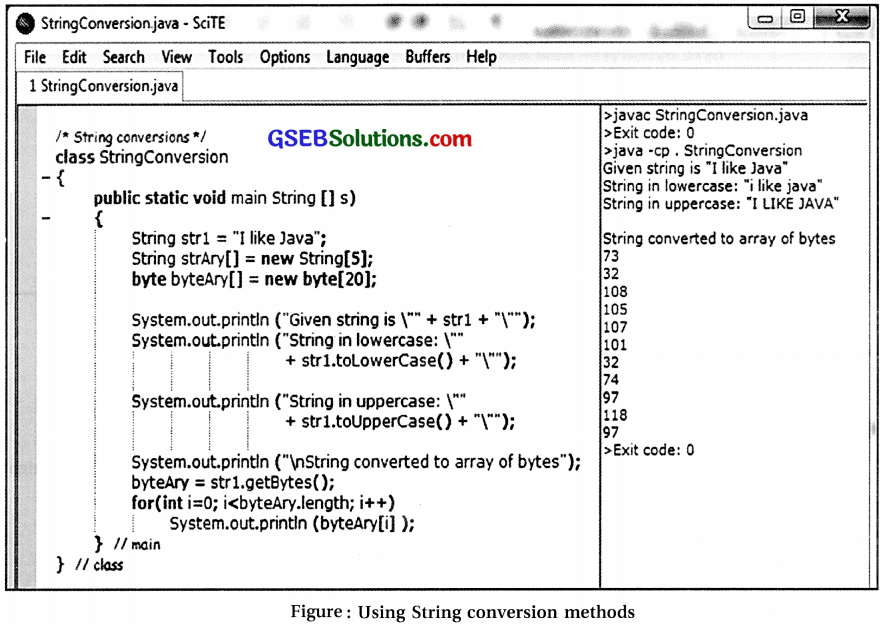• Note : With array variable, length is an attribute or property of array; whereas with String object length is a method. So there is a need to use ( ) while using length method with String object.
• String class of Java does not provide any method to reverse a string.
• Refer figure, where code listing is to reverse a string.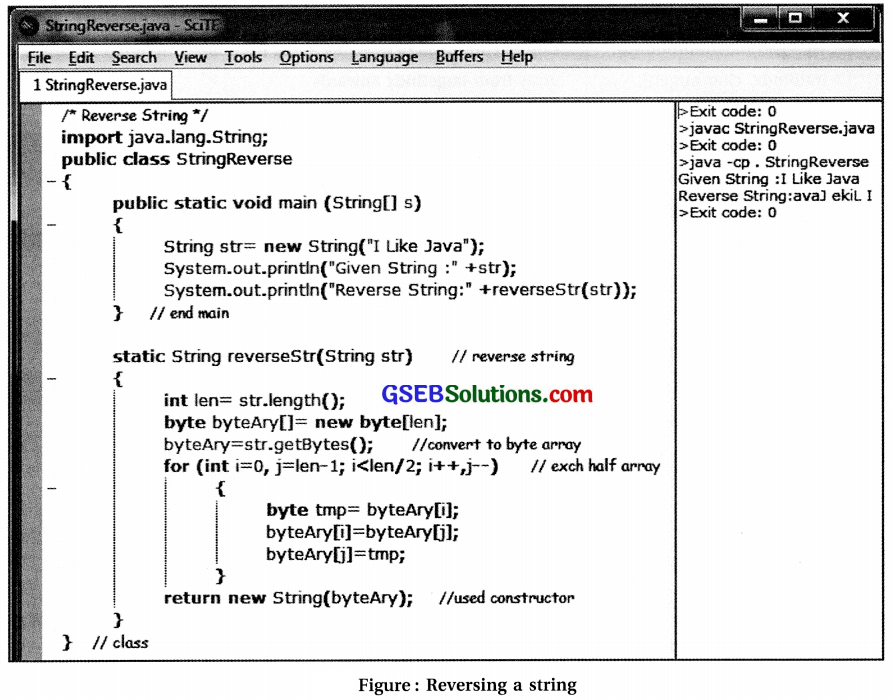• Below is a user-defined method reverseStr that reverses that string as follows :
1. Determine the length of string using length() method of String class.
2. Convert a string into an array of bytes using method getBytes() of String class.
3. Exchange half of the elements on left (starting from first towards right) with half of the elements on right (starting from last towards left) of a byte array.
4. Construct a String object from a byte array constructor and return it.
1.Date class

• Java library also provides Date class in java.util package.
• Date class encapsulates both date and time and represents the value using milliseconds precision.
• Figure shows the use of Date class with code listing and its output.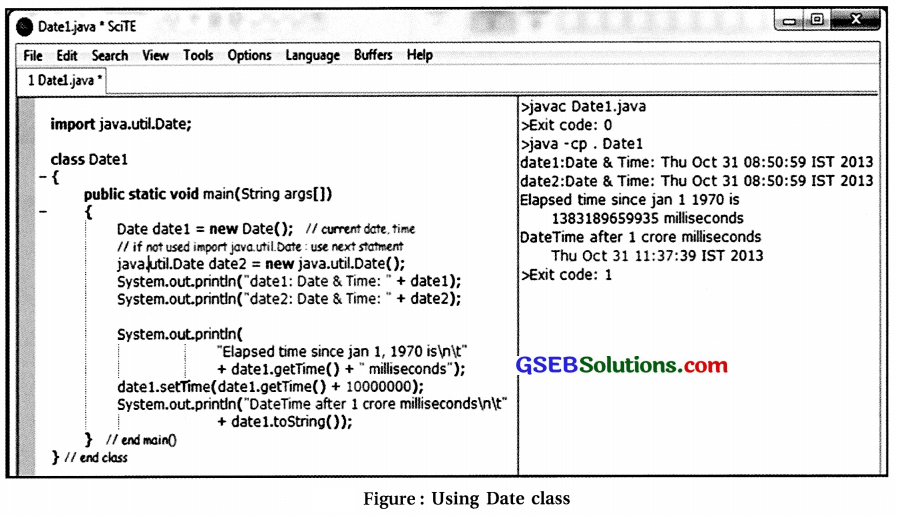• Table lists some of the methods of Date class.
 Method Description Date() Constructs Date object using current system time Date(long elapsed Time) Constructs Date object using specified time in milliseconds elapsed since January 1, 1970 GMT String toString() Returns a string representing date and time of invoking object long getTime() Returns number of milliseconds since January 1, 1970 GMT void setTime (long elapsed Time) Sets new date and time of an object using elapsed time

Table : Methods of Date classCalendar class

• Like Date class, Calendar class is also provided in java.util package.
• This class can be used to extract calendar information like year, month, date, hour, minute and second.
• Figure shows an example program to use Calender along with its output.
• Note the use of user-defined class method displayO used to display various components of date and time.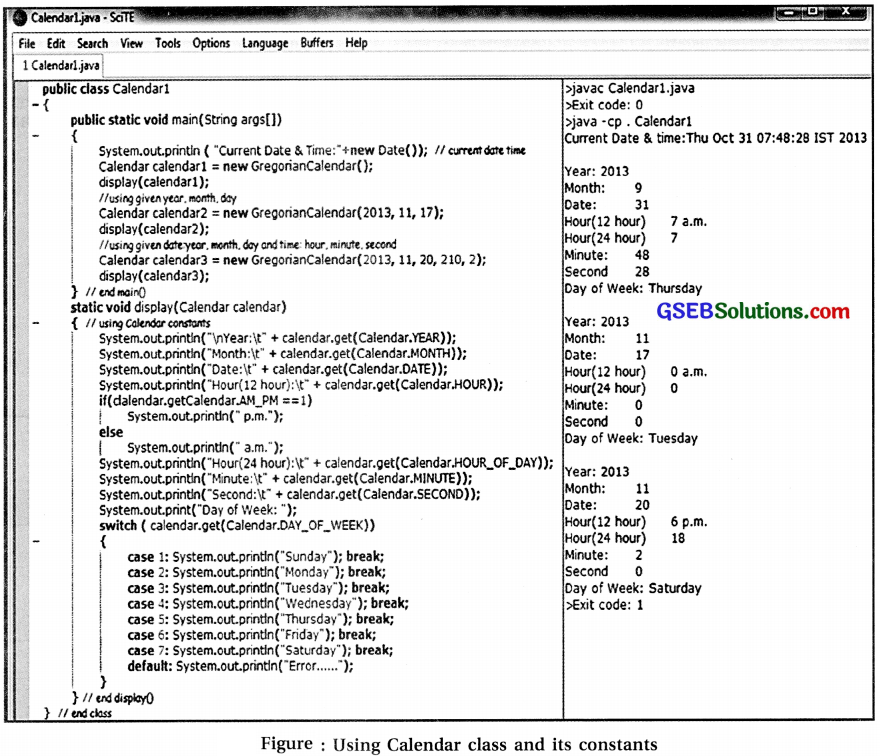• Like get methods, set methods are used to set the value of the field constants of Calendar class.
• For example, if calendar is an object of class Calender, then execution of call ‘calendar.set (Calendar.DATE, 20)’ will set the date to 20.
• Tablelists the integer constants defined in calendar class. These constants are given meaningful and self-explanatory names.
 Constant Description ‘ YEAR Year of calendar MONTH Month of Calendar (0 for January, 11 for December) DATE Day of Calendar month DAY_OF_MONTH Same as DATE HOUR Hour in 12-hour notation H 0 UR_0 F_D AY Hour in 24-hour notation MINUTE Minute SECOND Second AM_PM 0 for AM, 1 for PM DAY_OF_WEEK Day number within a week (1 for Sunday, 7 for Saturday) WEEK_OF_MONTH Week number within the month WEEK_OF_YEAR Week number within the year DAY_OF_YEAR Day number in the year (1 for the first day)

Table : Constants defiiwd in Calendar class

Question 4.
Choose the most appropriate option from those given below :

1) Which of the following refer to the starting index value in arrays ?
(A) 0
(B) 1
(C) null
(D)A11 of these
(A) 0

2) What is the size of second dimension in an array sales   ?
(A) 5
(B) 12
(C) 60
(D) 10
(B) 123) What will expression sales.length return for an array sales   ?
(A) 5
(B) 12
(C) 60
(D) 120
(C) 60

4) When an array sales   is declared without specifying initial values, what is the initial value of sales   ?
(A) 0
(B) default value
(C) compilation error
(D) 60
(C) compilation error

5) What does ‘length’ refer to for an object of String class ?
(A) Attribute
(B) Method
(C) Class variable
(D) Class name
(B) Method

6) If ‘str’ is the object of String class and its content is “Thank GOD”, then what is the value of str.lengthO ?
(A) 9
(B) 10
(C) 8
(D) 11
(A) 9

7) What type of value is returned when we use get method of Calendar class with constant DAY OF WEEK as an argument ?
(A) int
(B) char
(C) string
(D) boolean
(A) int

### Computer Class 12 GSEB Notes Chapter 9 Working with Array and String

Strings

• String is nothing but a sequence of characters. So 1-D array of characters can be considered as a string.
• As mentioned in earlier chapter, string literals are where a sequence of characters is enclosed between double quotes.
• To use variables that can store strings, Java supports two types of strings that are handled by two classes namely ‘String’ and ‘StringBuffer’.
• Some of the constructors that can be used to create an object are as follows :
1. String () without arguments create a String object with no character.
2. String (char ary[ ]) creates a String object with its initial value using ary argument.
3. String (char ary[ ], int start, int len) creates a String object using 1-D ary argument starting at aryfstart] with len number of characters.
4. String (String strObj) creates a String object which is same as object specified in argument.
5. String (string literal) creates a String object that refers to the literal specified in argument.
• Figure shows the use of various types of String class constructors. Here new operator has to be used while creating.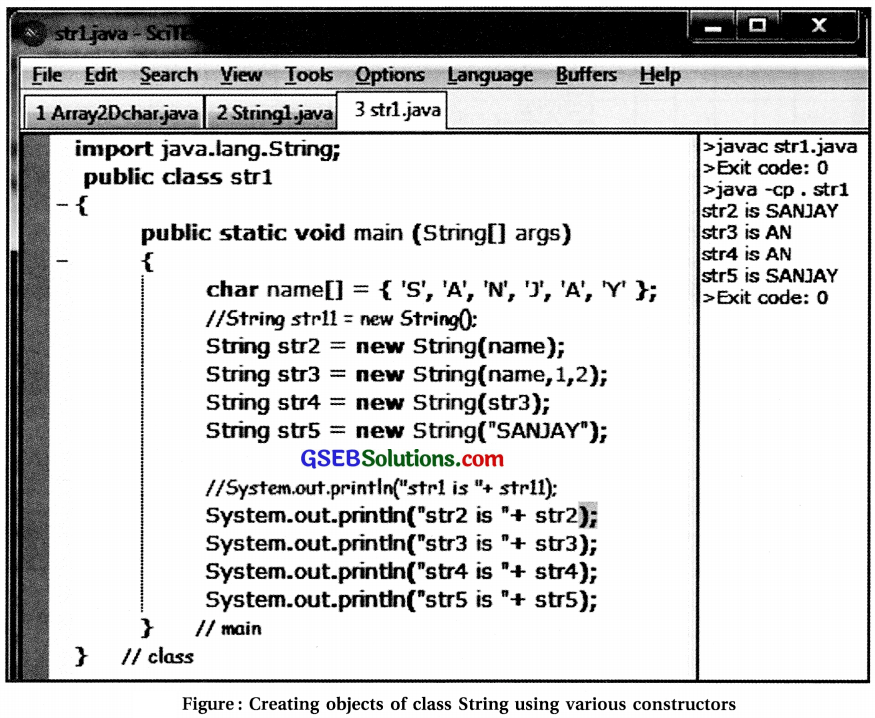• In Java, characters are stored using two bytes.
• To save space, if the characters are ASCII, an array of bytes should be used instead of array of characters.
• A constructor can be used with array of byes as an argument.
• It can be tried in the same program by replacing char array with byte array.
• Note : Literals are stored in memory. When two String objects are created using same string literals, memory space is not allocated for second object. Both objects refer to same memory location.
• Separate memory is allocated when string objects are created using new operator even if strings are identical.
• Code listing given in figure shows such an example :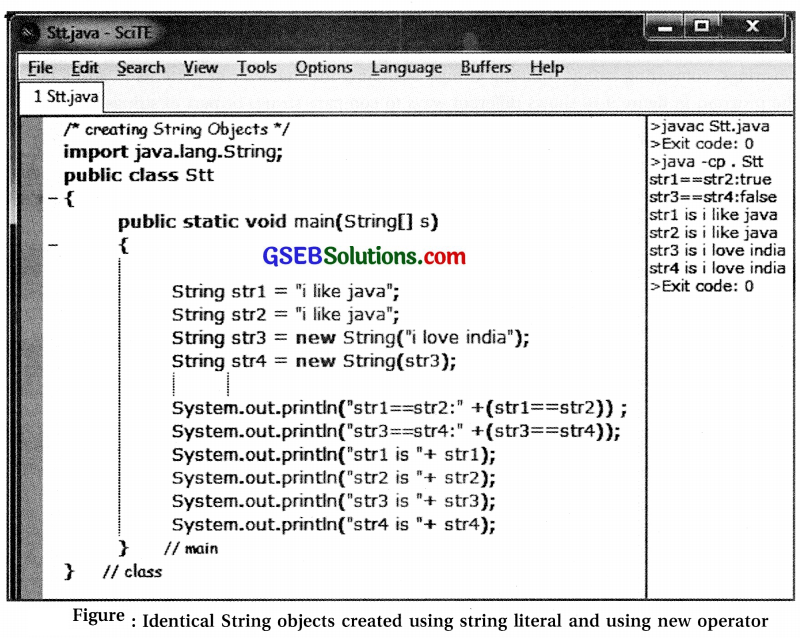• In code listing given in figure, note that “strl = = str2” compares the contents of strl and str2 and not the objects at strl and str2.
• String objects strl and str2 are created using same string literal but without using new operator.
• Here, both reference variables strl and str2 refer to the same instance as created for strl.
• For object str2, separate memory is not allocated as seen in figure.
• In the same program, String objects str3 and str4 are created using new operator.
• The contents of both these objects are same and they refer to different memory locations.
• Separate memory is allocated for these two objects as seen in figure.